# Evolutionary Computation Seminar Ch 16 19 Evolutionary Computation

• Slides: 25
Download presentation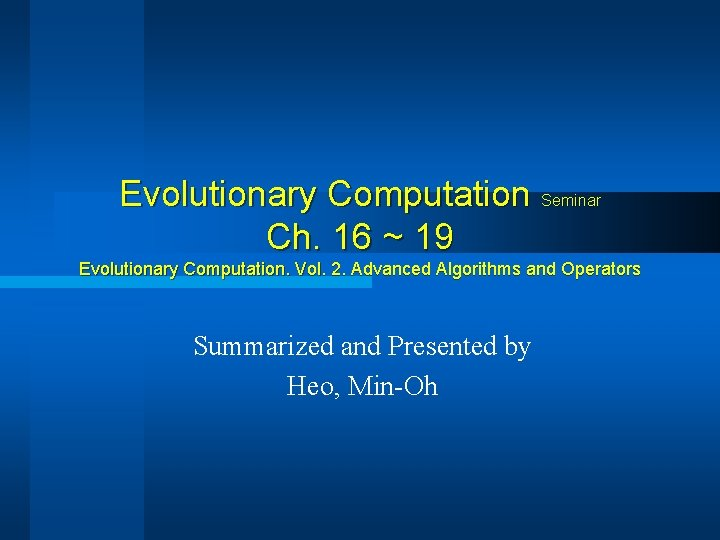Evolutionary Computation Seminar Ch. 16 ~ 19 Evolutionary Computation. Vol. 2. Advanced Algorithms and Operators Summarized and Presented by Heo, Min-Oh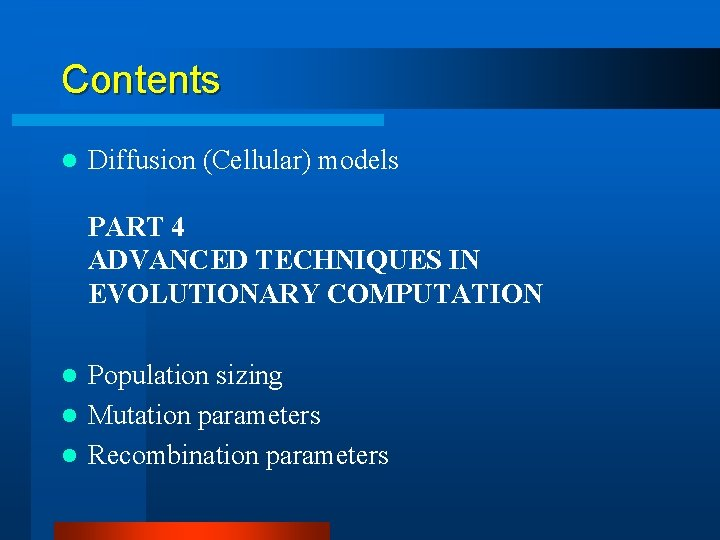Contents l Diffusion (Cellular) models PART 4 ADVANCED TECHNIQUES IN EVOLUTIONARY COMPUTATION Population sizing l Mutation parameters l Recombination parameters l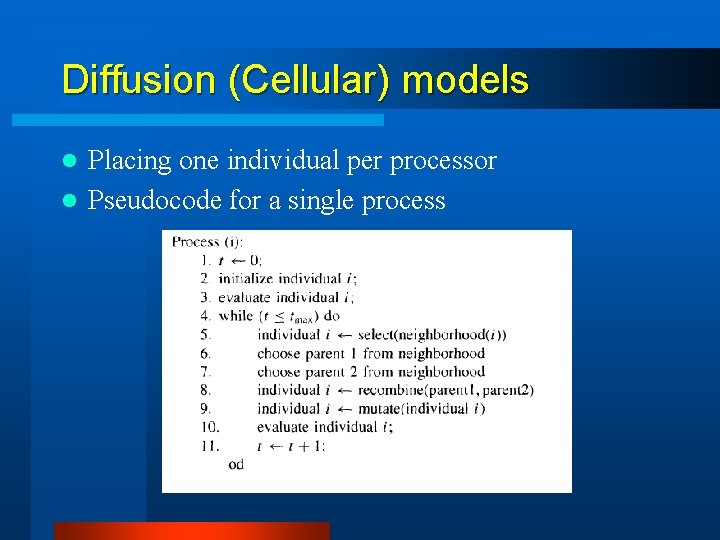Diffusion (Cellular) models Placing one individual per processor l Pseudocode for a single process l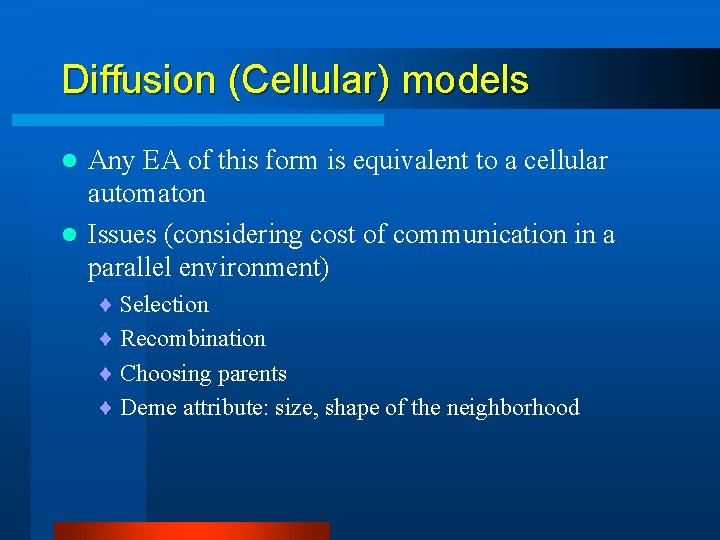Diffusion (Cellular) models Any EA of this form is equivalent to a cellular automaton l Issues (considering cost of communication in a parallel environment) l ¨ Selection ¨ Recombination ¨ Choosing parents ¨ Deme attribute: size, shape of the neighborhood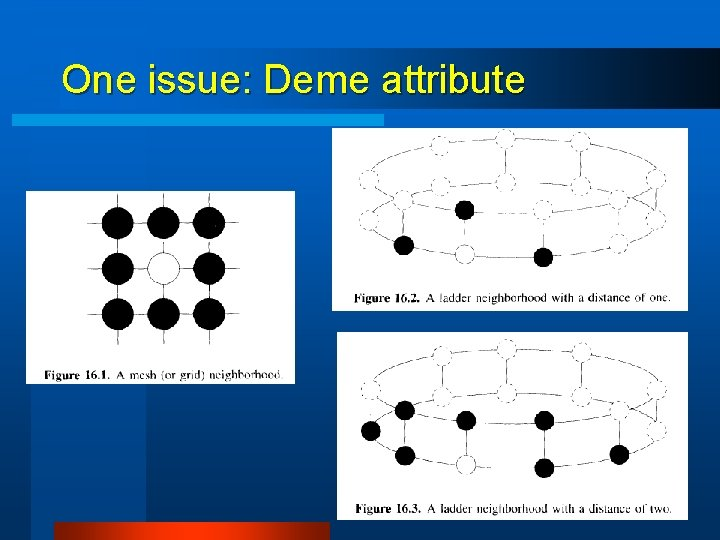One issue: Deme attribute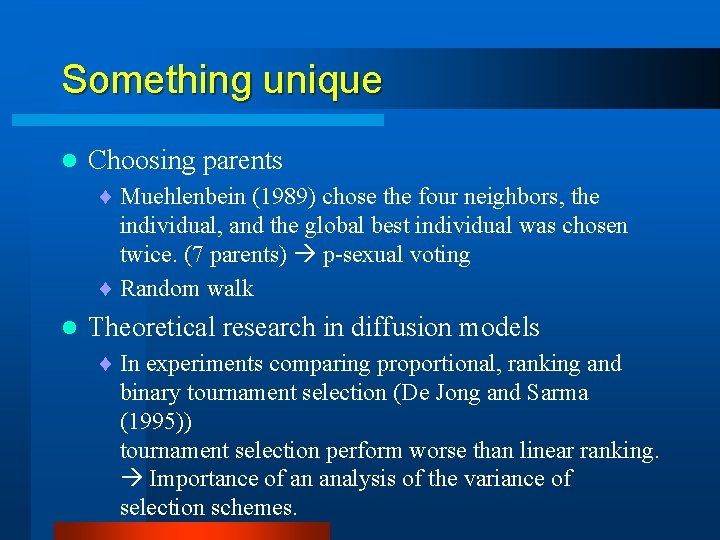Something unique l Choosing parents ¨ Muehlenbein (1989) chose the four neighbors, the individual, and the global best individual was chosen twice. (7 parents) p-sexual voting ¨ Random walk l Theoretical research in diffusion models ¨ In experiments comparing proportional, ranking and binary tournament selection (De Jong and Sarma (1995)) tournament selection perform worse than linear ranking. Importance of an analysis of the variance of selection schemes.PART 4 ADVANCED TECHNIQUES IN EVOLUTIONARY COMPUTATION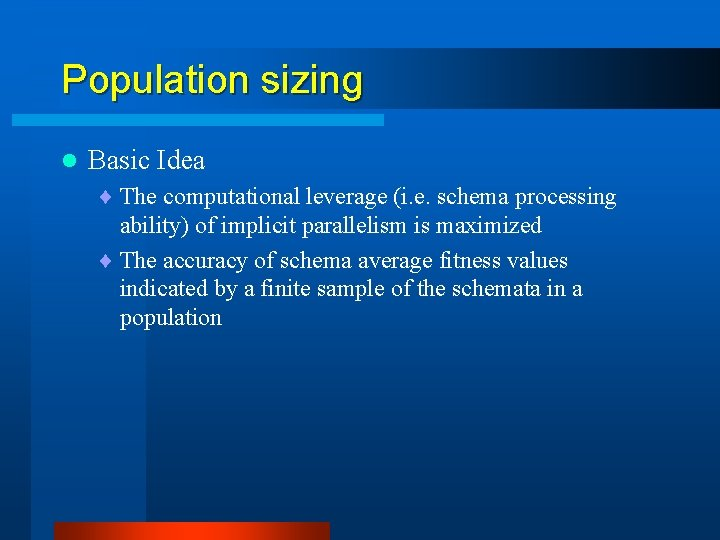Population sizing l Basic Idea ¨ The computational leverage (i. e. schema processing ability) of implicit parallelism is maximized ¨ The accuracy of schema average fitness values indicated by a finite sample of the schemata in a population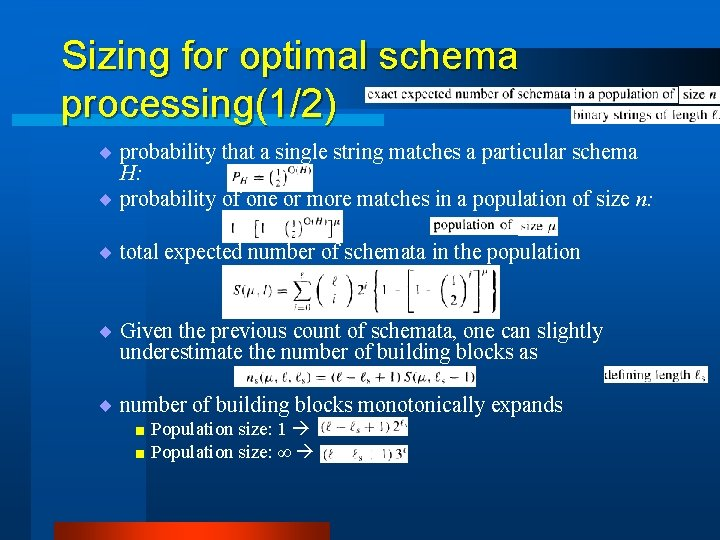Sizing for optimal schema processing(1/2) ¨ probability that a single string matches a particular schema H: ¨ probability of one or more matches in a population of size n: ¨ total expected number of schemata in the population ¨ Given the previous count of schemata, one can slightly underestimate the number of building blocks as ¨ number of building blocks monotonically expands size: 1 < Population size: ∞ < Population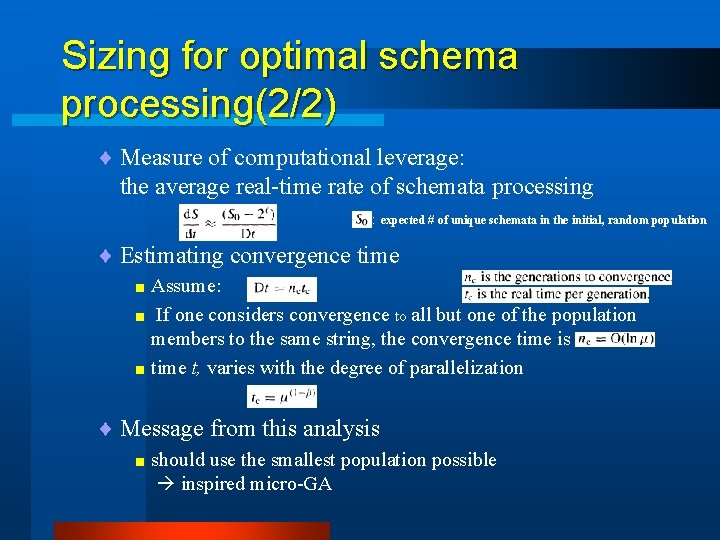Sizing for optimal schema processing(2/2) ¨ Measure of computational leverage: the average real-time rate of schemata processing : expected # of unique schemata in the initial, random population ¨ Estimating convergence time < Assume: If one considers convergence to all but one of the population members to the same string, the convergence time is < time t, varies with the degree of parallelization < ¨ Message from this analysis < should use the smallest population possible inspired micro-GASizing for accurate schema sampling (1/3) l Optimal population size for schema processing rate may not be the optimal size for ultimate GA effectiveness. Sampling error in small populations l variance of average fitness values of these schemata exists due to the various combinations of bits that can be placed in the ‘don’t care’ positions. : Collateral noise ¨ If one assumes f (H 1) > f (H 2) , there is a probability that fo(H 1) < fo(H 2) occur error ¨ By central limit thm, fo –values follows normal distribution with mean f (H) and variance σ2/n(H) ¨ Error probability (fo(H 1) < fo(H 2) is α) : f(): Average fitness values for schema fo (): observed fitness values for schema n (): number of copies for schema l setting n(H 1) and n(H 2) such that the error probability is lowered below the desired level. raising n(H) ‘sharpens’ the associated normal dist.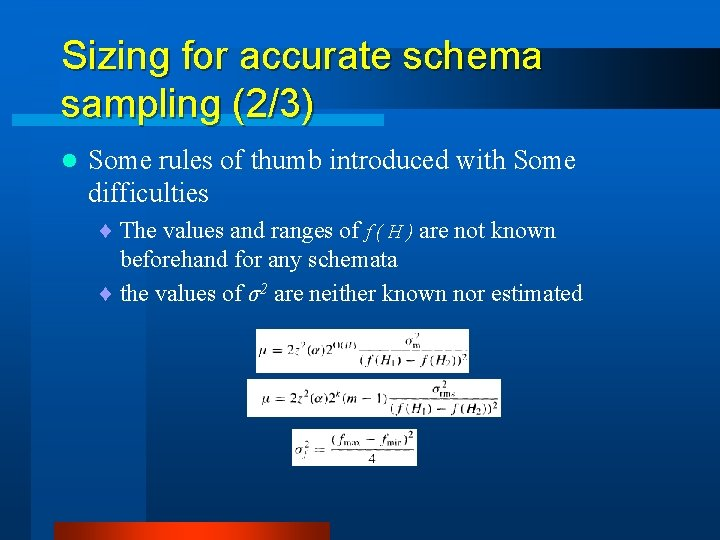Sizing for accurate schema sampling (2/3) l Some rules of thumb introduced with Some difficulties ¨ The values and ranges of f ( H ) are not known beforehand for any schemata ¨ the values of σ2 are neither known nor estimated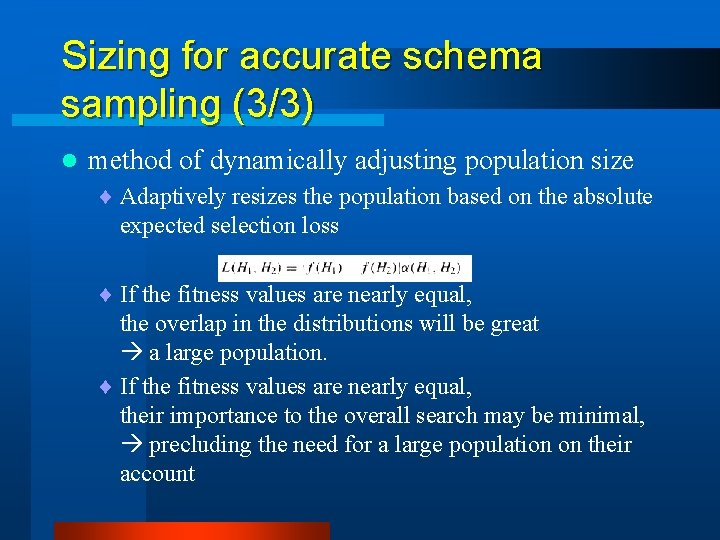Sizing for accurate schema sampling (3/3) l method of dynamically adjusting population size ¨ Adaptively resizes the population based on the absolute expected selection loss ¨ If the fitness values are nearly equal, the overlap in the distributions will be great a large population. ¨ If the fitness values are nearly equal, their importance to the overall search may be minimal, precluding the need for a large population on their account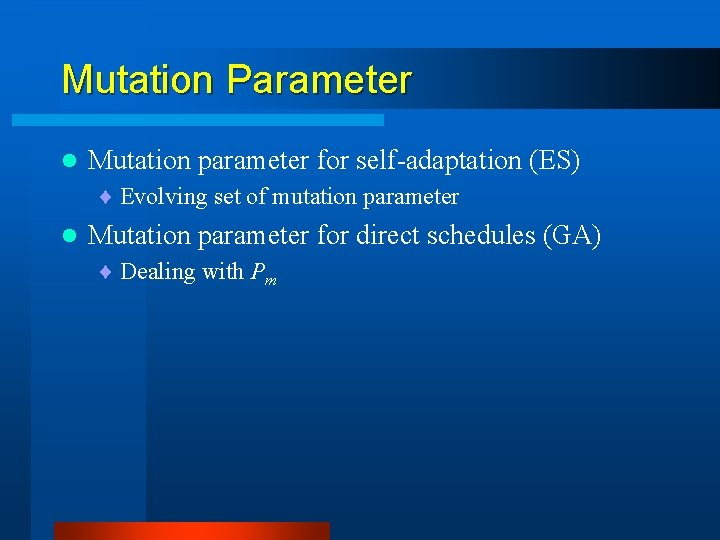Mutation Parameter l Mutation parameter for self-adaptation (ES) ¨ Evolving set of mutation parameter l Mutation parameter for direct schedules (GA) ¨ Dealing with Pm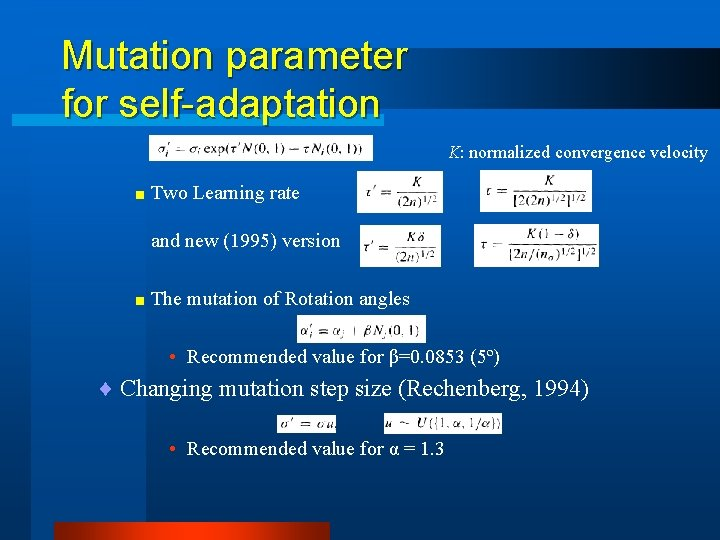Mutation parameter for self-adaptation K: normalized convergence velocity < Two Learning rate and new (1995) version < The mutation of Rotation angles • Recommended value for β=0. 0853 (5º) ¨ Changing mutation step size (Rechenberg, 1994) • Recommended value for α = 1. 3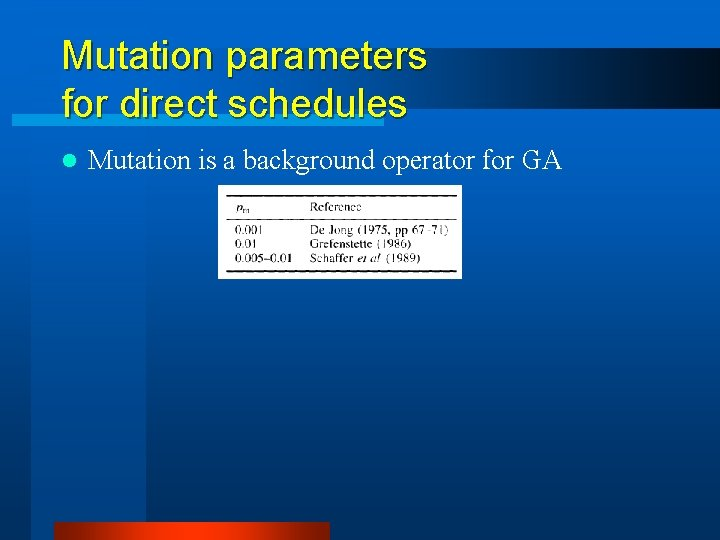Mutation parameters for direct schedules l Mutation is a background operator for GA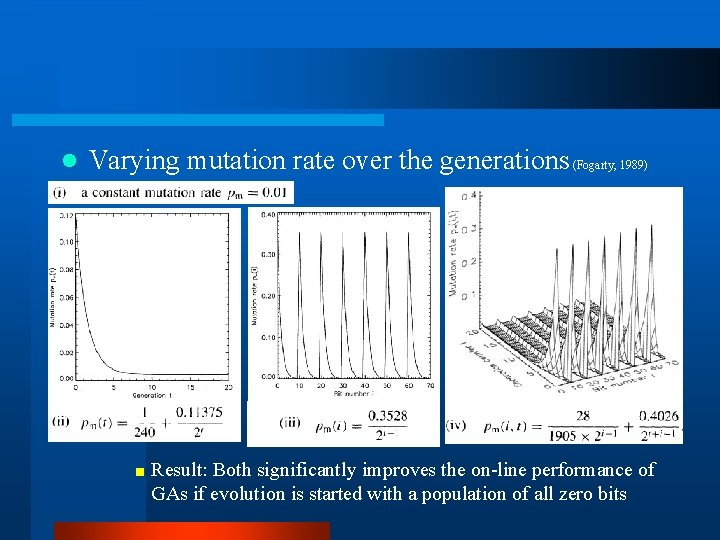l Varying mutation rate over the generations (Fogarty, 1989) < Result: Both significantly improves the on-line performance of GAs if evolution is started with a population of all zero bits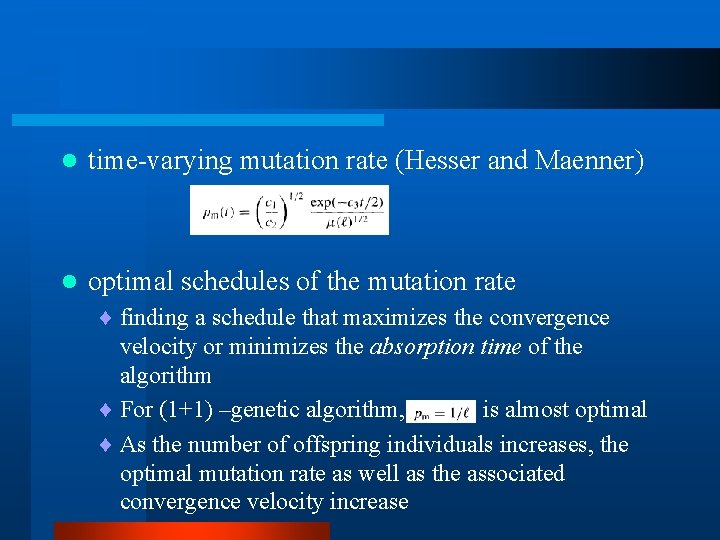l time-varying mutation rate (Hesser and Maenner) l optimal schedules of the mutation rate ¨ finding a schedule that maximizes the convergence velocity or minimizes the absorption time of the algorithm ¨ For (1+1) –genetic algorithm, is almost optimal ¨ As the number of offspring individuals increases, the optimal mutation rate as well as the associated convergence velocity increaseNondeterministic schedules for controlling the 'amount‘of mutation t/T=0. 2, b=5 t/T=0. 6, b=5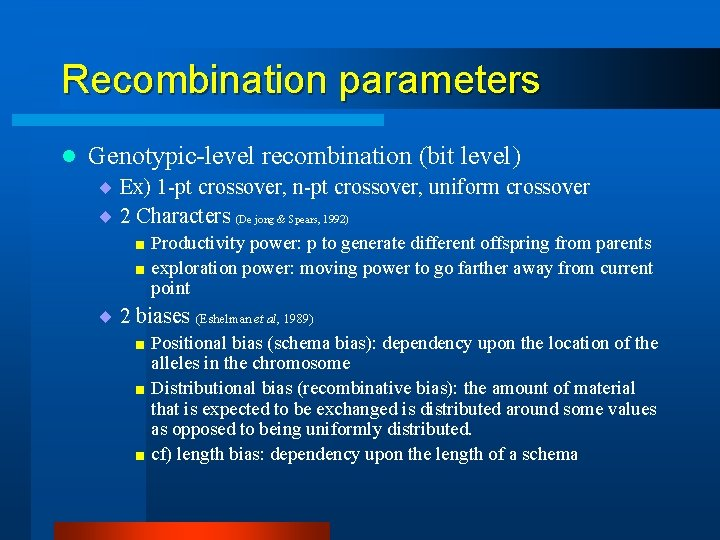Recombination parameters l Genotypic-level recombination (bit level) ¨ Ex) 1 -pt crossover, n-pt crossover, uniform crossover ¨ 2 Characters (De jong & Spears, 1992) < Productivity power: p to generate different offspring from parents < exploration power: moving power to go farther away from current point ¨ 2 biases (Eshelman et al, 1989) < Positional bias (schema bias): dependency upon the location of the alleles in the chromosome < Distributional bias (recombinative bias): the amount of material that is expected to be exchanged is distributed around some values as opposed to being uniformly distributed. < cf) length bias: dependency upon the length of a schema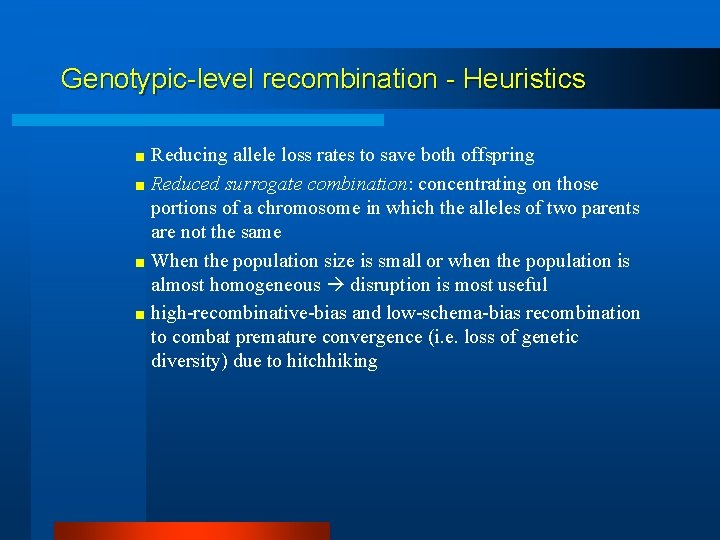Genotypic-level recombination - Heuristics < Reducing allele loss rates to save both offspring < Reduced surrogate combination: concentrating on those portions of a chromosome in which the alleles of two parents are not the same < When the population size is small or when the population is almost homogeneous disruption is most useful < high-recombinative-bias and low-schema-bias recombination to combat premature convergence (i. e. loss of genetic diversity) due to hitchhikingPhenotypic-level recombination (problem specific) ¨ Some difficult cases < Hamming cliffs: large changes in the binary encoding are required to make small changes to the real values ¨ Real-valued representation < EA, ES < Interval schemata ¨ Representation for permutation or ordering problemsControl of recombination parameters ¨ Static techniques < assume that one particular recombination operator should be applied at some static rate for all problems ¨ Predictive techniques < designed to predict the performance of recombination operators < Computing the past performance of an operator as an estimate of the future performance of an operator ¨ Adaptive techniques < Recognize when bias is correct or incorrect, and recover from incorrect biases when possible < Tag-based: attach extra information to a chromosome, which is both evolved by the EA and used to control recombination < Rule-based: adapt recombination using control mechanisms and data structures that are external to the EA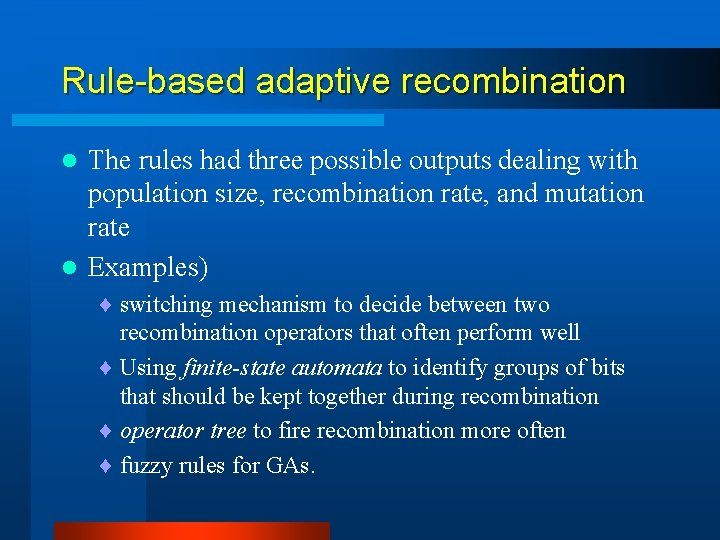Rule-based adaptive recombination The rules had three possible outputs dealing with population size, recombination rate, and mutation rate l Examples) l ¨ switching mechanism to decide between two recombination operators that often perform well ¨ Using finite-state automata to identify groups of bits that should be kept together during recombination ¨ operator tree to fire recombination more often ¨ fuzzy rules for GAs.